gemseo / core

# discipline_data module¶

Provide a dict-like class for storing disciplines data.

class gemseo.core.discipline_data.DisciplineData(data, input_to_namespaced=None, output_to_namespaced=None)[source]

Bases: MutableMapping

A dict-like class for handling disciplines data.

This class replaces a standard dictionary that was previously used for storing discipline data. It allows handling values bound to pandas.DataFrame as if they were multiple items bound to numpy.ndarray. Then, an object of this class may be used as if it was a standard dictionary containing numpy.ndarray, which is the assumption made by the clients of the MDODiscipline subclasses.

As compared to a standard dictionary, the methods of this class may hide the values bound to pandas.DataFrame and instead expose the items of those latter as if they belonged to the dictionary.

If a dict-like object is provided when creating a DisciplineData object, its contents is shared with this latter, such that any changes performed via a DisciplineData object is reflected into the passed in dict-like object.

If a DisciplineData is created from another one, their contents are shared.

A separator, by default ~, is used to identify the keys of the items that are bound to an array inside a pandas.DataFrame. Such a key is composed of the key from the shared dict-like object, the separator and the name of the target column of the pandas.DataFrame. When such a key is queried, a numpy.ndarray view of the pandas.DataFrame column is provided.

Printing a DisciplineData object prints the shared dict-like object.

Nested dictionaries are also supported.

Examples

>>> from gemseo.core.discipline_data import DisciplineData
>>> import numpy as np
>>> import pandas as pd
>>> data = {
...         'x': 0,
...         'y': pd.DataFrame(data={'a': np.array()}),
...     }
>>> disc_data = DisciplineData(data)
>>> disc_data['x']
0
>>> disc_data['y']
a
0  0
>>> # DataFrame content can be accessed with the ~ separator.
>>> disc_data['y~a']
array()
>>> # New columns can be inserted into a DataFrame with the ~ separator.
>>> disc_data['y~b'] = np.array()
>>> data['y']['b']
0    1
Name: b, dtype: int64
>>> # New DataFrame can be added.
>>> disc_data['z~c'] = np.array()
>>> data['z']
c
0  2
>>> type(data['z'])
<class 'pandas.core.frame.DataFrame'>
>>> # DataFrame columns can be deleted.
>>> del disc_data['z~c']
>>> data['z']
Empty DataFrame
Columns: []
Index: 
>>> # Iterating is only done over the exposed keys.
>>> list(disc_data)
['x', 'y~a', 'y~b']
>>> # The length is consistent with the iterator.
>>> len(disc_data)
3

Parameters:
• data (MutableData) – A dict-like object or a DisciplineData object.

• input_to_namespaced (NamespacesMapping) – The mapping from input data names to their prefixed names.

• output_to_namespaced (NamespacesMapping) – The mapping from output data names to their prefixed names.

clear() None.  Remove all items from D.
copy()[source]

Create a shallow copy.

Returns:

The shallow copy.

Return type:

DisciplineData

get(k[, d]) D[k] if k in D, else d.  d defaults to None.
items() a set-like object providing a view on D's items
keys() a set-like object providing a view on D's keys
pop(k[, d]) v, remove specified key and return the corresponding value.

If key is not found, d is returned if given, otherwise KeyError is raised.

popitem() (k, v), remove and return some (key, value) pair

as a 2-tuple; but raise KeyError if D is empty.

setdefault(k[, d]) D.get(k,d), also set D[k]=d if k not in D
update([E, ]**F) None.  Update D from mapping/iterable E and F.

If E present and has a .keys() method, does: for k in E: D[k] = E[k] If E present and lacks .keys() method, does: for (k, v) in E: D[k] = v In either case, this is followed by: for k, v in F.items(): D[k] = v

values() an object providing a view on D's values
SEPARATOR = '~'

The character used to separate the shared dict key from the column of a pandas DataFrame.

## Examples using DisciplineData¶Create a surrogate discipline

Create a surrogate disciplinePlug a surrogate discipline in a Scenario

Plug a surrogate discipline in a Scenario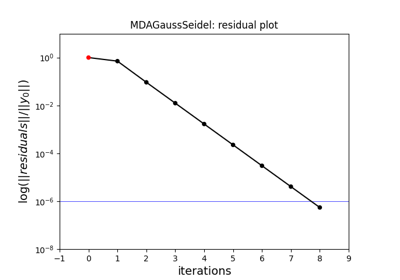Gauss-Seidel MDA

Gauss-Seidel MDA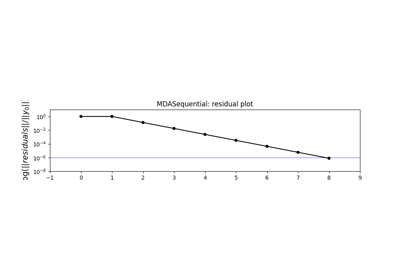Hybrid Jacobi/Newton MDA

Hybrid Jacobi/Newton MDA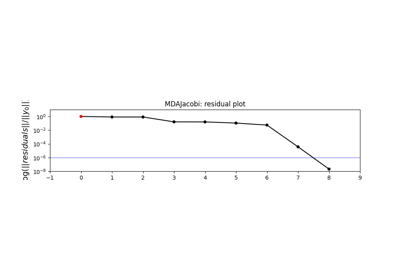Jacobi MDA

Jacobi MDA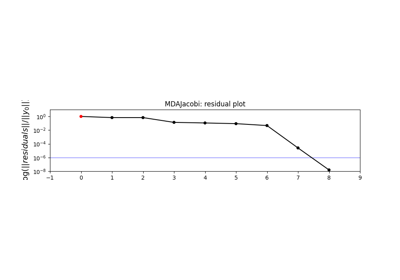MDAChain

MDAChainMDAChain with independent parallel MDAs

MDAChain with independent parallel MDAs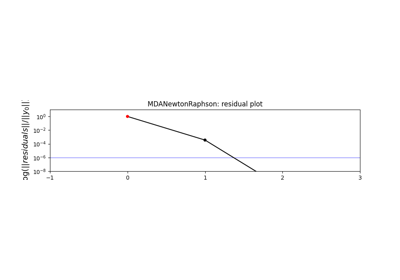Newton-Raphson MDA

Newton-Raphson MDA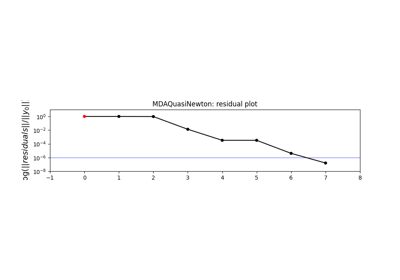Quasi-Newton MDA

Quasi-Newton MDAMDA

MDACreate a discipline from analytical expressions

Create a discipline from analytical expressions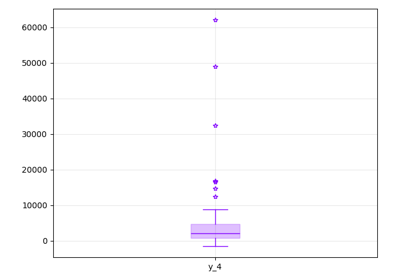Empirical estimation of statistics

Empirical estimation of statistics